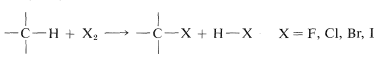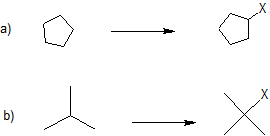# 5.11: Reactivity and Selectivity

$$\newcommand{\vecs}{\overset { \rightharpoonup} {\mathbf{#1}} }$$ $$\newcommand{\vecd}{\overset{-\!-\!\rightharpoonup}{\vphantom{a}\smash {#1}}}$$$$\newcommand{\id}{\mathrm{id}}$$ $$\newcommand{\Span}{\mathrm{span}}$$ $$\newcommand{\kernel}{\mathrm{null}\,}$$ $$\newcommand{\range}{\mathrm{range}\,}$$ $$\newcommand{\RealPart}{\mathrm{Re}}$$ $$\newcommand{\ImaginaryPart}{\mathrm{Im}}$$ $$\newcommand{\Argument}{\mathrm{Arg}}$$ $$\newcommand{\norm}{\| #1 \|}$$ $$\newcommand{\inner}{\langle #1, #2 \rangle}$$ $$\newcommand{\Span}{\mathrm{span}}$$ $$\newcommand{\id}{\mathrm{id}}$$ $$\newcommand{\Span}{\mathrm{span}}$$ $$\newcommand{\kernel}{\mathrm{null}\,}$$ $$\newcommand{\range}{\mathrm{range}\,}$$ $$\newcommand{\RealPart}{\mathrm{Re}}$$ $$\newcommand{\ImaginaryPart}{\mathrm{Im}}$$ $$\newcommand{\Argument}{\mathrm{Arg}}$$ $$\newcommand{\norm}{\| #1 \|}$$ $$\newcommand{\inner}{\langle #1, #2 \rangle}$$ $$\newcommand{\Span}{\mathrm{span}}$$$$\newcommand{\AA}{\unicode[.8,0]{x212B}}$$

## Comparing Reactivity

Given the knowledge that a particular reaction will proceed at a suitable rate, a host of practical considerations are necessary for satisfactory operation. These considerations include interference by possible side reactions that give products other than those desired, the ease of separation of the desired product from the reaction mixture, and costs of materials, apparatus, and labor. We shall consider these problems in connection with the important synthetic reactions discussed in this book.

The chlorination of saturated hydrocarbons can be induced by light, but also can be carried out at temperatures of about $$300^\text{o}$$ in the dark. Under such circumstances the mechanism is similar to that of light-induced chlorination, except that the chlorine atoms are formed by thermal dissociation of chlorine molecules. Solid carbon surfaces catalyze thermal chlorination, possibly by aiding in the cleavage of the chlorine molecules.

Direct monohalogenation of saturated hydrocarbons works satisfactorily only with chlorine and bromine. For the general reactionthe calculated $$\Delta H^\text{0}$$ value is negative and very large for fluorine, negative and moderate for chlorine and bromine, and positive for iodine (see Table 4-7). With fluorine, the reaction evolves so much heat that it may be difficult to control, and products from cleavage of carbon-carbon as well as of carbon-hydrogen bonds may be obtained. The only successful, direct fluorination procedure for hydrocarbons involves diffusion of minute amounts of fluorine mixed with helium into liquid or solid hydrocarbons at low temperatures, typically $$-78^\text{o}$$ (Dry Ice temperature). As fluorination proceeds, the concentration of fluorine can be increased. The process is best suited for preparation of completely fluorinated compounds, and it has been possible to obtain in this way amounts of $$\left( CF_3 \right)_4C$$ and $$\left( CF_3 \right)_3 C-C \left( CF_3 \right)_3$$ from 2,2-dimethylpropane and 2,2,3,3-tetramethylbutane corresponding to $$10$$-$$15\%$$ yields based on the fluorine used.

Bromine generally is much less reactive toward hydrocarbons than chlorine is, both at high temperatures and with activation by light. Nonetheless, it usually is possible to brominate saturated hydrocarbons successfully. Iodine is unreactive.

Table: Calculated Heat of Reaction for Halogenation fo Hydrocarbons
Halogen (X) $$\Delta H^o$$ (kcal/mole)a
F -116
Cl -27
Br -10
I 13
aCalculated from the bond energies of Table 4-3.

The chlorination of methane does not have to stop with the formation of chloromethane (methyl chloride). It is usual when chlorinating methane to obtain some of the higher chlorination products: dichloromethane (methylene chloride), trichloromethane (chloroform), and tetrachloromethane (carbon tetrachloride):In practice, one can control the degree of substitution to a considerable extent by controlling the methane-chlorine ratio. For example, for monochlorination to predominate, a high methane-chlorine ratio is necessary such that the chlorine atoms react with $$CH_4$$ and not with $$CH_3Cl$$.

### Selectivity in Alkane Halogenation

For propane and higher hydrocarbons for which more than one monosubstitution product is generally possible, difficult separation problems bay arise when a particular product is desired. For example, the chlorination of 2-methylbutane $$3$$ at $$300^\text{o}$$ gives all four possible monosubstitution products. On a purely statistical basis, we may expect the ratio of products from 2-methylbutane to correlate with the number of available hydrogens at the various positions of substitution in the ratio 6:1:2:3 ($$50\%$$:$$8\%$$:$$17\%$$:$$25\%$$:). However, as can be seen from the strengths of bonds between hydrogen and primary, secondary, and tertiary carbons are not the same and we would expect the weaker $$C-H$$ bonds to preferentially react with $$Cl \cdot$$. As such, the proportion of the tertiary halide is about three times that expected on a statistical basis which is in accord with our expectation that the tertiary $$C-H$$ bond of 2-methylbutane should be the weakest of the $$C-H$$ bonds.Bromine atoms are far more selective than chlorine atoms. This is not unexpected becauseis endothermic, whereas corresponding reactions with a chlorine atoms usually are exothermic (data from Table 4-6). Bromine removes only those hydrogens that are relatively weakly bonded to a carbon atom. As predicted, attack of $$Br \cdot$$ on 2-methylbutane leads mostly to 2-bromo-2-methylbutane, some secondary bromide, and essentially no primary bromides:When the structure of the alkane is symmetrical, then the fast reactivity of chlorination can be used for efficiency. When the structure of the alkane can produce a range of monohalogenated products, then the selectivity of bromination can be used to produce the most stable product in the greatest percentage.

## Exercises

1. Specify the optimum halogenation conditions (Cl2/heat or Br2/heat) to produce the indicated major product.Solution

1.